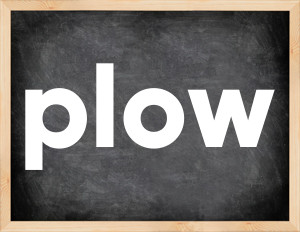# Plow past tenseThe English verb 'plow' is pronounced as [ˈplaʊ].
Related to: regular verbs.
3 forms of verb plow: Infinitive (plow), Past Simple - (plowed), Past Participle - (plowed).

## Here are the past tense forms of the verb plow

👉 Forms of verb plow in future and past simple and past participle.
❓ What is the past tense of plow.

## Plow: Past, Present, and Participle Forms

Base Form Past Simple Past Participle
plow [ˈplaʊ]

plowed [plowed]

plowed [plowed]

## What are the 2nd and 3rd forms of the verb plow?

🎓 What are the past simple, future simple, present perfect, past perfect, and future perfect forms of the base form (infinitive) 'plow'?

### Learn the three forms of the English verb 'plow'

• the first form (V1) is 'plow' used in present simple and future simple tenses.
• the second form (V2) is 'plowed' used in past simple tense.
• the third form (V3) is 'plowed' used in present perfect and past perfect tenses.

## What are the past tense and past participle of plow?

The past tense and past participle of plow are: plow in past simple is plowed, and past participle is plowed.

### What is the past tense of plow?

The past tense of the verb "plow" is "plowed", and the past participle is "plowed".

### Verb Tenses

Past simple — plow in past simple plowed (V2).
Future simple — plow in future simple is plow (will + V1).
Present Perfect — plow in present perfect tense is plowed (have/has + V3).
Past Perfect — plow in past perfect tense is plowed (had + V3).

### plow regular or irregular verb?

👉 Is 'plow' a regular or irregular verb? The verb 'plow' is regular verb.

## Examples of Verb plow in Sentences

•   You need it to plow a field. (Present Simple)
•   They plowed several sites (Past Simple)
•   I plowed through a book (Past Simple)
•   They used tractors to plow the field. (Past Simple)
•   Her face was rough, and ploughed with wrinkles. (Past Simple)
•   The car sped up, and ploughed into a fence. (Past Simple)
•   Farmer Jones plowed his east field last week. (Past Simple)
•   In this area you might plough up some valuables. (Present Simple)
•   I plowed into this company and doubled up my capital (Past Simple)
•   The ship plowed through the water. (Past Simple)

Along with plow, words are popular apologize and cake.

Verbs by letter: , , , , , , , , , , , , , , , , , , , , , , , , .